# Most common mistake in Depreciation

Change in life of assets has been specified in new companies act 2013. However it does not suggested change in depreciation method. Hence there is no need to recalculate effect of depreciation from the period of asset inception for depreciation. Change in the life of assets is only a change in accounting estimate but not a change in accounting policy. And only Net value of the asset lying in the books need to be depreciated for the remaining period of asset life as per AS-6 & AS-10. I like to explain how to calculate in reducing balance method due to this change.

The following formula can be used to calculate rate of depreciation for Reducing balance method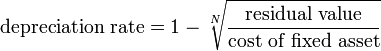However the above formula is based on following two assumptions

1. Residual value is always more than zero.
2. Depreciation is calculated at equal periodical ends.

The assumption 1 above can be overcome by putting One Rupee as residual value as it would not effect financial statements materially

However in case of assumption 2 above the following illustration method may be adopted although it was not accurate method to overcome material misstatements.

Date of asset capitalized and ready to use - 01.03.2015
Capitalized value of asset (in Rupees) - 1,00,000/-
Residual value (5%) - 5000/-
Life of asset - 3 years
Depreciation as per above method - 63.16%
No. of days asset depreciable in the financial year 2014-15 = 31
Hence Depreciation will be calculated as follows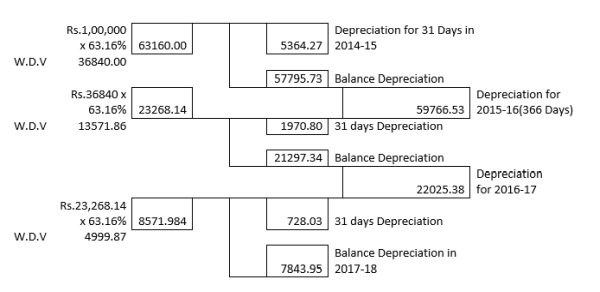Depreciation for the financial year 2014-15 = Rs.1,00,000/- x 63.16% x 31/365 = 5364.247

Depreciation for the financial year 2015-16 will be sum of the following two calculations

• Depreciation 1 = Rs.1,00,000/- x 63.16% x (365-31)/365
• Depreciation 2 = (Rs.1,00,000/- x (100%-63.16%)) x 63.16% x 31/366 = 1970.80

Hence total depreciation for financial year 2015-16 is = 57,795.73 + 1970.80 = 59,766.53

For the succeeding periods may also be calculated as indicated above.

If we calculate depreciation on Net asset value on 31.03.2015 (i.e Rs.1,00,000 – 5364.247 = 94,635.7530) @ 63.16% directly then at the end of life of the asset you will get more than Rs.5000/-. And such deviation from residual required will depend on date of capitalization of asset we will examine in the following cases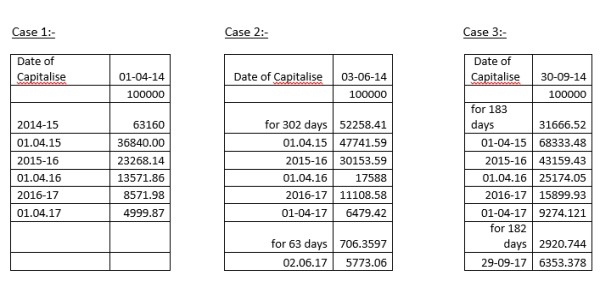We get maximum deviation when asset is capitalized on 30-09.14.For any asset capitalised on 30.09.14 we will get maximum deviation in the residual value because it was the date which got maximum number of days of distance from either financial year beginning date or financial year ending date. This is due to change in the value at end of financial year on which rate of depreciation is applied.

However I know how to resolve this problem which will be explained as below. Companies Act 2013 doesn`t specified the rate of deprecition but the life of asset. Hence we can calculate the appropriate rate for depreciation calculation in a method other than by using the formula specified in the first page of this article. We can get the rate appropriate rate by two methods

Method 1 :-

Consider if asset is capitalized on 03-06-14

Using 63.16% deviation we got from residual value = 5773.06 -5000 = 773.06
Using 70% deviation we will get = 1670.20 (calculated)

Hence required rate of depreciation

= 63.16 + (773.06*(70-63.16)/(773.06+1670.20))
= 63.16+ 2.1642
= 65.3242%

In this method we will loose accuracy depending on number of digits we use after decimal points. This is the method generally used to calculate Internal Rate of Return and this concept also used in formula for finding mode in Quantitative Techniques subject.

However I prefer Method 2 which will be explained in below :

In this method I use Microsoft Excel sheet for calculation which produces maximum accuracy possible than above method 1 . Observe the below picture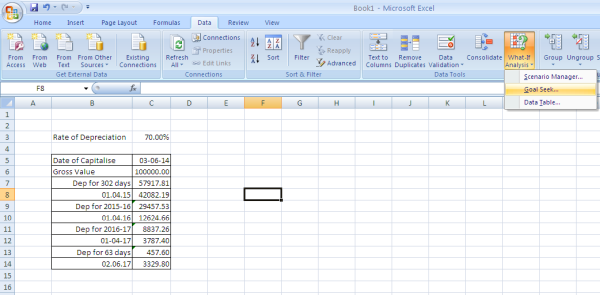In the above picture I have calculated depreciation @ 70% for the asset capitalized on 03-06-14

Step 1:- While calculating depreciation by formula for the purpose of rate I have made reference to Cell Number C3 every time I have calculated.

Step 2:- After making calculation by formula as specified in above step we will get the values shown in picture, Then use the option called “What if Analysis” and in that “Goal Seek” we will get this get this option in Tools of 2003 version also and the version used in the above is 2007.

Step3:- We will get the following picture and specify the values as shown in the picture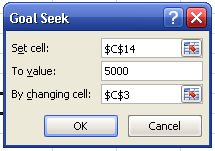In the above picture I got \$C\$14 by selecting C14 cell. I have specified value of 5000 assuming that 5% of 1,00,000 is residual value. I got \$C\$3 by selecting C3 cell. Then press ok Then it will recalculate and modify the rate to 65.07% which most accurate method that can be used by everybody

In case of old assets the following may be done

Take W.D.V value on 31.03.2014 as per books which is arrived by calculating in old rates specified in Companies Act 1956 in the place of Rs.100000/- specified in the above example. Then calculate it for the remaining period of life and arrive at residual value. And then apply the above specified method 2 for more accuracy and get the required rate of depreciation.

However in some cases old assets remaining useful life will not be exactly in years but can also be some days or months in addition to complete years. In such cases also the method, I have preferred will work but not the formula specified in the first page of this article.

Let see how the formula specified in the first page has derived

Basing on the assumptions of the W.D.V at the end of 1st year will be as below
W.D.V = (100% - R%)XC

Where R = Rate of Depreciation; C = Cost of the asset
X = Multiply Symbol

W.D.V at the end of 2nd year will be as below
W.D.V = (100% - R%)2XC

W.D.V at the end of Nth year will be as below
W.D.V = (100% - R%)NXC

However at the end of Nth year it will be at Residual Value, hence
(100% - R%)NXC = Residual Value

• (100% - R%)N = Residual Value/C
• 100% - R% = (Residual Value/C)1/N
• R% = 100% - (Residual Value/C)1/N

Which is nothing butIf an asset like Buildings with RCC Frame structure having life 60 Years, it may lengthy procedure to arrive residual value following above.To arrive at residual value in less steps for any assets the following steps may be followed

Step 1
Calculate W.D.V for the first year in normal way (i.e Rs.57917.81 in diagram explained in Excel above)

Step 2
(W.D.V of Step 1)(100% - R%)(N-1)

Step 3

Depreciation that will be provided in last financial year = (Out come of Step 2)(R%)(No of days of life in last financial of asset/No of Days in that financial Year)

Residual Value = Out come of Step 2 (Less) Depreciation to be provided in last financial year

In the above calculation R% is rate of depreciation and “N” is life of asset in years

The author can also be reached atcaravikishore@rediffmail.com

Tags :

Category Accounts
Other Articles by -
Kishore

Report Abuse

### Popular Articles#### Extension of Income Tax and GST Due Dates amid Second Wave of COVID-19 - Need of the hour?#### TDS deducted? Be careful if you are filing ITR only with Form 26AS#### New Tax Regime#### Tax Compliance and Statutory due dates for the m/o May, 2021#### Relief Measures declared by CBIC and CBDT in Indirect Tax and Direct Tax#### Compliance for Private Limited Companies under new MCA regime#### Tax Planning tips for individuals & HUF for FY 2021-22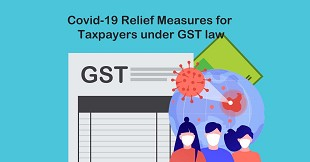#### Covid-19 Relief Measures for Taxpayers under GST law

CCI Articles